# Linear equations

## Summary and examples

An example of a linear equation is: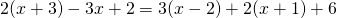and the question is to calculate the value ofthat makes the equation an identity. We call this solving the equation.

To solve this equation, we proceed as follows.

1. First, we remove the parentheses: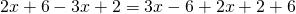2. Then, in the left- and right-hand side we gather the's and constants: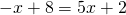3. Now we bring the's to the left-hand side and the constants to the right-hand side: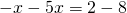resulting in: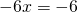4. We divide both sides of the equation by the coefficientof, in this case by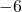. Then we get: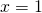which is the solution of the equation.

It is possible that this procedure leads to an equation of the form: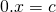Then there are two possibilities.

1.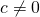In this case no value ofcan satisfy the equation. The left-hand side always equals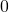, while the right-hand side is not equal to. The equation has no solution.

2.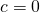Now we have the equation: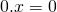and any value ofsatisfies the equation.

##### Example 1

Solve: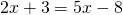We can write this equation as: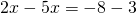so:and thus: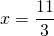##### Example 2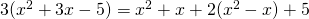We can write this equation as:or: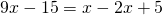or: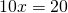and thus: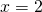Note that at first sight the equation seems a quadratic equation. However, the squares ofdisappear, with a linear equation as a result.

##### Example 3

Solve: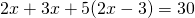We can write this equation as: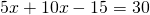or: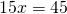and thus is the solution: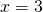##### Example 4

Solve: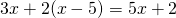We can write this equation as: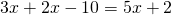or: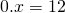No value ofcan satisfy this equation and thus the equation has no solution.

##### Example 5

Solve: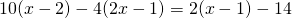We can write this equation as: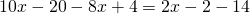or:or:Any value ofsatisfies this equation. This equation has an infinite number of solutions.

0